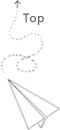# algorithm_bigO_notation.md

Typical notataion about the algorithm complexity and some thoughts

when consider a specific optimization, it might makes sense if it decrease the upper bound, otherwise, the optimization in the constant item maybe meaningless. When considering the optimization for a system, it might makes sense if you consider it from the complexity aspects.

### big theta notation

When we use big-Θ notation, we’re saying that we have an asymptotically tight bound on the running time. “Asymptotically” because it matters for only large values of nnn. “Tight bound” because we’ve nailed the running time to within a constant factor above and below.

### big O notation

big-omega, asymptotic upper bound, It would be convenient to have a form of asymptotic notation that means “the running time grows at most this much, but it could grow more slowly.” We use “big-O” notation for just such occasions.

For example, although the worst-case running time of binary search is big theta (log_2n), it would be incorrect to say that the binary search runs in big theta (log_2n), sometimes, we could find suitable value at first guess, the running time will be less then the (log_2n). Therefore, we could use the big O(log_2n) to represent this scenario.

We may much care about the upper bound for most algorithms.

### big Omega notation

We use big-Ω notation for asymptotic lower bounds, since it bounds the growth of the running time from below for large enough input sizes.